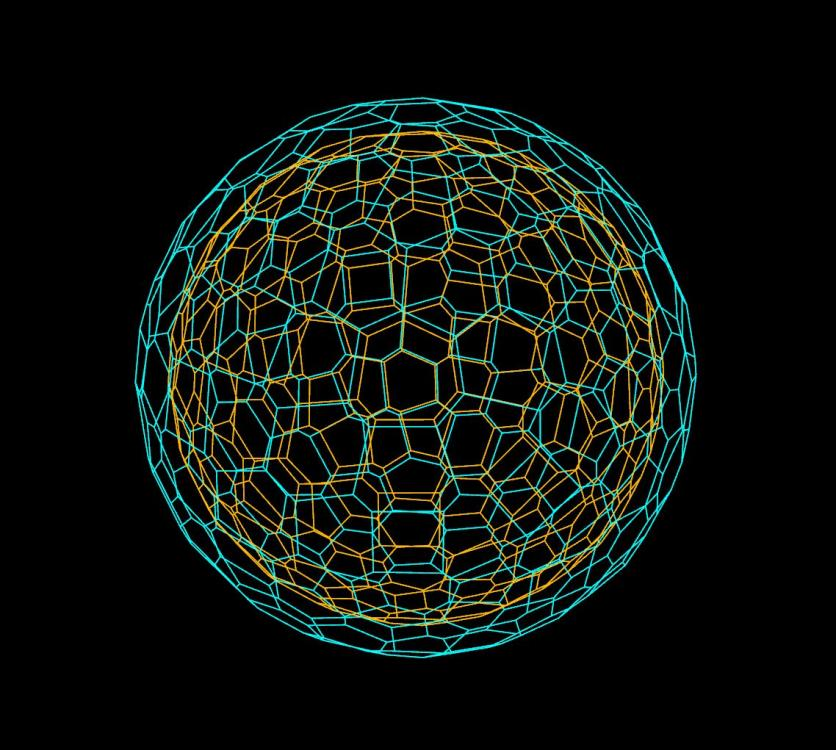# Create lines between 2 geos with the same point count and number

## Recommended Posts

Hi there,

I'm studying VEX and at the moment I'm trying to create polylines lines between 2 spheres, one sphere is the copy transform of the first and scaled down.

After dissecting the problem, I have the possible steps in my head but cannot quite transfer it to VEX yet.

I'm thinking, gather the point numbers from the first input (0) of the Attribute Wrangle and store on a variable. Do the same with the point numbers from the second input of the Attribute Wrangle node (1). I'm not sure if an array is needed in here as I'm still learning VEX. Then for each point number, create a line between the point number from the first input to the respective point number from the second input as the @ptnum attribute serves as pointid. For this to happen, both geos have to have the same topology and or point number and I'm my case I got that already.

I'm attaching a reference image for the scene i'm working on.

That said, how can one create lines between 2 geos with the exact same topology and point number? Ideally, the lines would start from the same point number on the first geo and end on the same point number on the second geo?

I've found some implementations on the internet using the nearpoints function but this approach wouldn't connect the exact same points between the two geos. I'm attaching an image as reference to illustrate what I'm talking about. Damn, VEX is no jokeAny thoughts would be appreciated.##### Share on other sites

Hi Rival,

here are some ways to connect copied points by their ID. Use the enumerate SOP to give each point an individual ID before copying.

Non-VEX:
Add SOP: Polygons > By group > By attribute: id

H-Script + VEX:
Grid set to rows:

```// HScript expressions for grid resolution
nuniquevals('../copy1', D_POINT, 'id')
nuniquevals('../copy1', D_PRIMITIVE, 'copynum')```
```// point wrangle for connecting
int index = vertexprimindex(0, i@vtxnum);
int pt_crv = findattribval(1, 'point', 'id', i@primnum, index);
v@P = point(1, 'P', pt_crv);```

VEX:

```// detail wrangle
int num = nuniqueval(0, 'point', 'id');
for(int i = 0; i < num; i++){
int pts[] = {};
int count = findattribvalcount(0, 'point', 'id', i);
for(int k = 0; k < count; k++){
int pt = findattribval(0, 'point', 'id', i, k);
append(pts, pt);
}
int prim_crv = addprim(0, 'polyline', pts);
setprimgroup(0, 'connect', prim_crv, 1, 'set');
}```

nuniqueval() returns how many IDs exist in total
findattribvalcount() returns the number of points that share the same ID
findattribval() returns the point number of each member with the same ID

connect_ids.hiplc

•3
##### Share on other sites

• 4 weeks later...

That is exactly what I was trying to achieve. Thank you for sharing the code snippets, much appreciated!

## Join the conversation

You can post now and register later. If you have an account, sign in now to post with your account.
Note: Your post will require moderator approval before it will be visible.×   Pasted as rich text.   Paste as plain text instead

Only 75 emoji are allowed.

×   Your previous content has been restored.   Clear editor

×   You cannot paste images directly. Upload or insert images from URL.

×
×